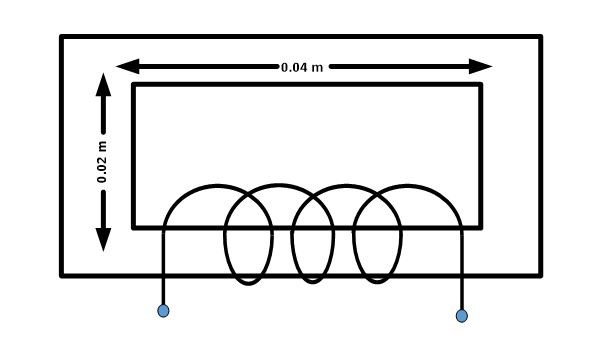# Magnetic Field Intensity | Definition Formula

Want create site? Find Free WordPress Themes and plugins.

Magnetomotive force, ℑ , per unit length, is called the magnetic field intensity H.

## Magnetic Field Intensity Unit

Magnetic field intensity is also known as the magnetizing force which is measured is ampere-turns per meter (A-t/m).

Of primary concern, however, is the magnetomotive force needed to establish a certain flux density, B in a unit length of the magnetic circuit.

## Magnetic Field Intensity Formula

The letter symbol for magnetizing force (magnetic field intensity) is H. The following relationship defines H as;

$H=\frac{\mathfrak{F}}{l}$

Where

ℑ =applied MMF in ampere-turns

l =average length of the magnetic path in meters

Example

Find the magnetic field intensity in the magnetic circuit shown below:Solution:

We can calculate the intensity using following formula:

$H=\frac{F}{l}=\frac{(2.5*{{10}^{2}})*(1.5*{{10}^{-1}})}{1.2*{{10}^{-1}}}$

$H=\frac{3.75*{{10}^{1}}}{1.2*{{10}^{-1}}}=3.125*{{10}^{2}}A-t/m$

If the dimensions of the magnetic path were changed, the value of H would also change. Fox example, if the total length of the magnetic path doubled, we should expect the value of H to decrease to one-half its previous amount.

If physical dimensions are double for the above circuit, then magnetizing force will be;

$H=\frac{3.75*{{10}^{1}}}{2.4*{{10}^{-1}}}=1.5625*{{10}^{2}}A-t/m$

So, we can observe that, for a given number of ampere-turns, the magnetizing force varies inversely per unit length of the magnetic path.